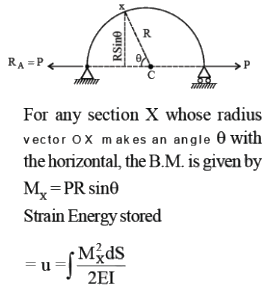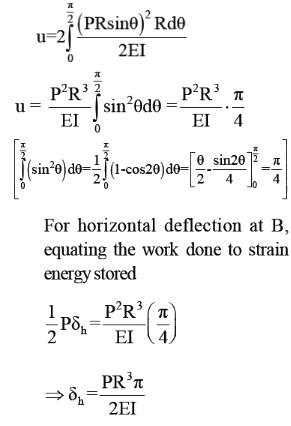Courses

# Strength Of Materials

## 25 Questions MCQ Test GATE Mechanical (ME) 2022 Mock Test Series | Strength Of Materials

Description
This mock test of Strength Of Materials for GATE helps you for every GATE entrance exam. This contains 25 Multiple Choice Questions for GATE Strength Of Materials (mcq) to study with solutions a complete question bank. The solved questions answers in this Strength Of Materials quiz give you a good mix of easy questions and tough questions. GATE students definitely take this Strength Of Materials exercise for a better result in the exam. You can find other Strength Of Materials extra questions, long questions & short questions for GATE on EduRev as well by searching above.
QUESTION: 1

### A mild steel tube 4m long, 30mm, internal diameter and 4mm thick is used as a strut with both ends hinged. Collapsing load is:- Take E = 2.1 × 105 N/mm2

Solution: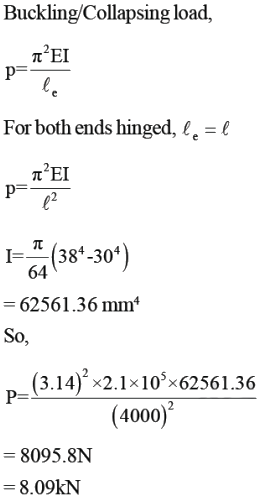QUESTION: 2

### A strut 2.5m long is 60mm in  diameter. One end of the strut is fixed while its other end is hinged. Using factor of safety 3.5, the safe compressive load for the member is: E = 2.1 × 105 N/mm2

Solution: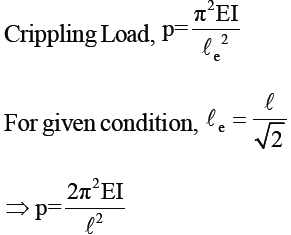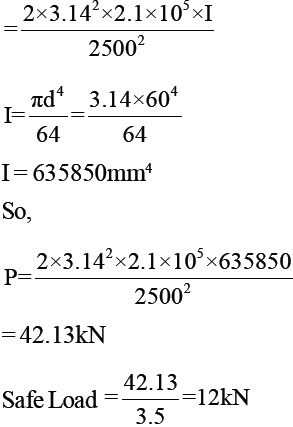QUESTION: 3

### Figure shows a slender bar of area A and length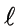pinned to immovable supports. Rise in temperature required to produce buckling of bar is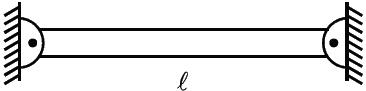Solution: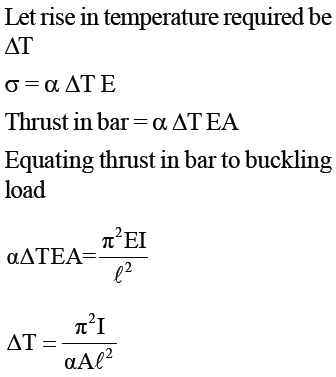QUESTION: 4

A solid circular compression member 50mm in diameter is to be replaced by a hollow circular section of the same material. Find the size of hollow section if internal diameter is 0.6 times external diameter.

Solution: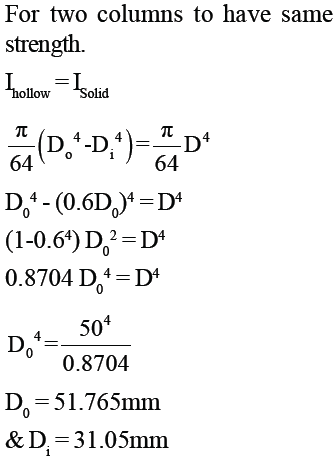QUESTION: 5

A hollow alloy tube 5m with external and internal diameters equal to 40mm and 25mm respectively was found to extend by 6.4mm under a tensile load of 60kN. When tube is used as column with both ends pinned, find  safe compressive load for tube. (FOS = 4)

Solution: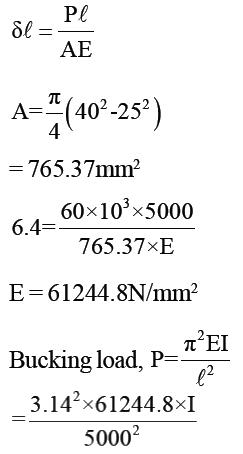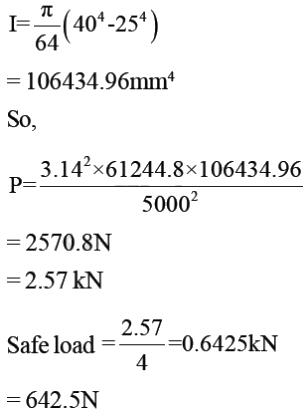QUESTION: 6

Find the greatest length of mild steel rod 25mm × 25mm which can be used as compression member with one end fixed and the other end free to carry a working load of 25 kN.
Factor of safety = 4,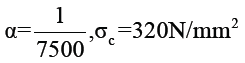Solution: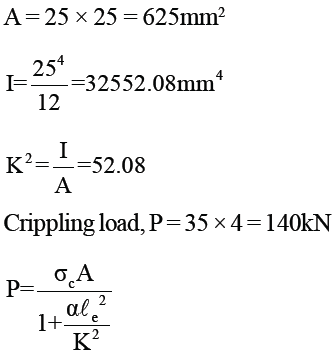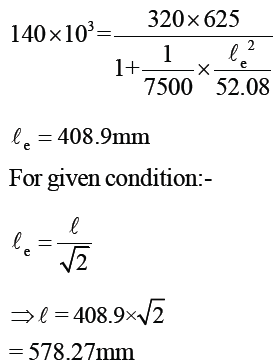QUESTION: 7

Hollow C.I. column whose outside diameter is 200mm has thickness of 20mm. It is 7.5m long and is fixed at both ends. Find the ratio of Euler’s and Rankine’s critical loads. For C.I., αc = 550N/mm2, α = 1/1600, E = 8 × 10N/mm2

Solution: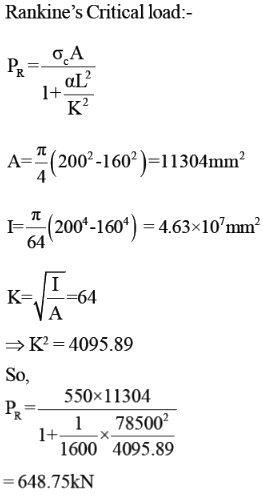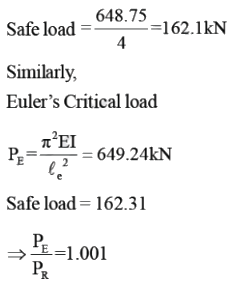QUESTION: 8

Common data for 8 & 9

Beam 8m long fixed at both ends carries a UDL over whole span. Take EI = 9.5 × 109 kNmm. Find load intensity on beam:-

Q. When maximum Bending Moment should not exceed 40 kN-m.

Solution: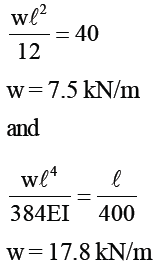QUESTION: 9

Common data for 8 & 9

Beam 8m long fixed at both ends carries a UDL over whole span. Take EI = 9.5 × 109 kNmm. Find load intensity on beam:-

Q. When maximum deflection should not exceed 1/400 of the span.

Solution:
QUESTION: 10

Vessel in the shape of spherical shell 800mm in diameter, 10mm shell thickness is completely filled with a fluid at atmospheric pressure. Additional fluid is then pumped in till pressure increases by 5 N/mm2.

Find volume of this additional fluid.

μ = 0.25, E = 2 × 105 N/mm2

Solution: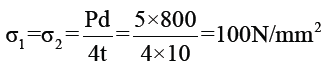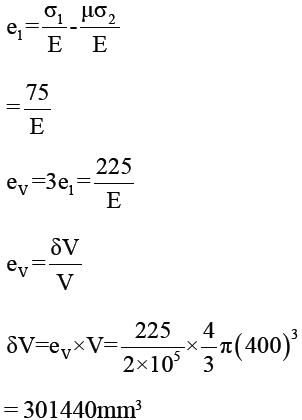QUESTION: 11

A solid shaft of 200mm diameter has the same cross-sectional area as that of hollow shaft of same material with inside diameter of 150mm. Find ratio of power transmitted by two shafts at same speed.

Solution: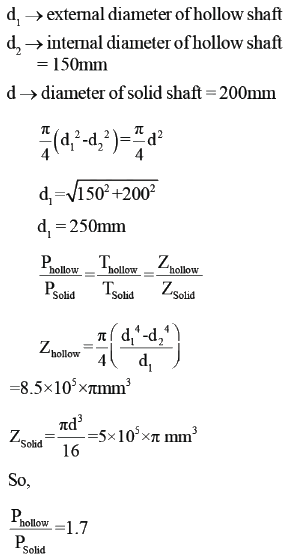QUESTION: 12

A hollow shaft is to transmit 300kW at 80rpm. If shear stress is not to exceed 60 N/mm2 and internal diameter is 0.6 of the external diameter, find external and internal diameters assuming maximum torque is 1.4 times the
mean torque.

Solution: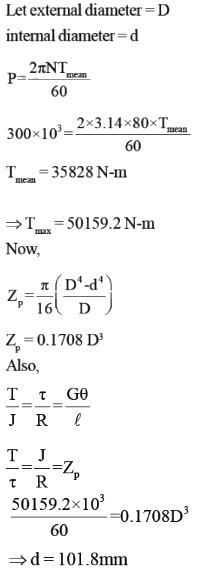QUESTION: 13

A cast iron test beam 20mm × 20mm in section and 1m long and supported at the end fails when a central  load of 640 N is applied. What uniformily distributed load will break a cantilever of the same material 50mm wide, 100mm deep and 2m long?

Solution: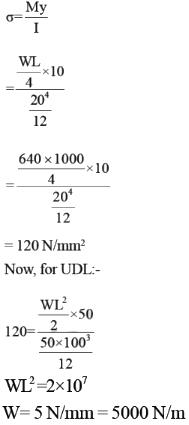QUESTION: 14

An I-section beam as shown, is simply supported over a span of 10m. If the maximum permissible bending stress is 80 N/mm2. What concentrated load can be carried at a distance of 3.5m from one support.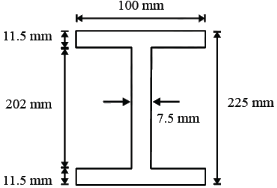Solution: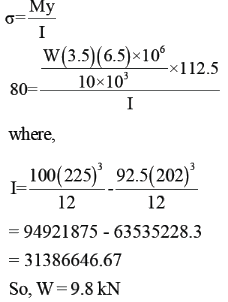QUESTION: 15

A 3m high pole stands as a vertical cantilever fixed at its base. It has to support a horizontal load of 10kN
at its top. Find the minimum diameter required if the pole is of wood, if the permissible bending stress is 15N/mm2

Solution: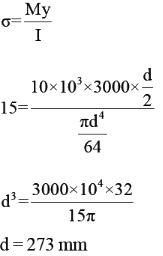QUESTION: 16

A steel beam of solid circular section 25mm in diameter and length 1m is simply supported at both ends, and is loaded by its own weight only. If steel weights at 77000 N/m3, what is the length of the beam at which it just starts yeilding.

Take Yeild stress = 207N/mm2

Solution: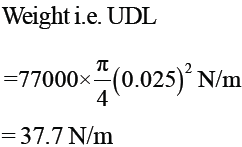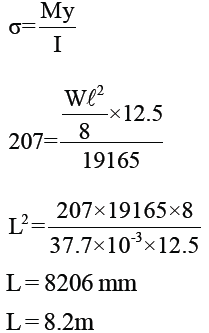QUESTION: 17

Two planes AB and BC which are at right angles carry shear stresses of intensity 17.5 N/mm2 while these
planes also carry tensile stress of 70 N/mm2 and compressive stress of 35N/mm2 respectively. Position of
principal planes is given as:-

Solution: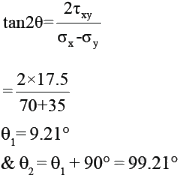QUESTION: 18

Consider 2 - D state of stress as shown:-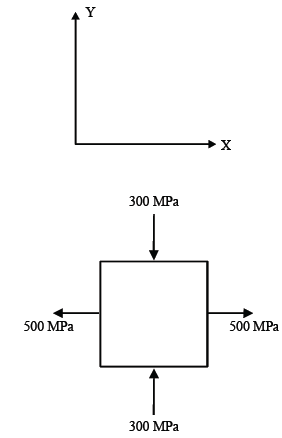Coordinates of centre of Mohr’s circle is:-

Solution: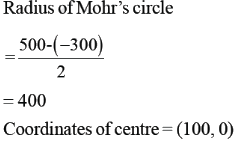QUESTION: 19

At a point in a 2D stress system, σx = 100 N/mm2, σy =τxy 40 N/mm2. What is the radius of Mohr’s circle
for stress drawn with scale 1cm= 10 N/mm2 ?

Solution: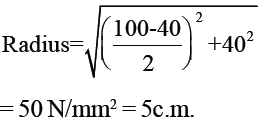QUESTION: 20

A steel bar 15mm in diameter is pulled axially by a force of 10 kN. If the bar is 250mm long, calculate the strain energy per unit volume stored by bar in (N-mm/mm3)

(Esteel = 2 × 105 N/mm2)

Solution: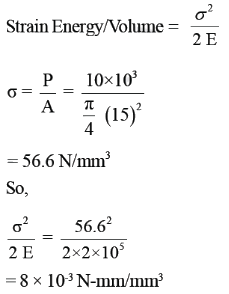QUESTION: 21

Rod with coefficient of linear expansion 0.000012 per oC and E = 1 × 105 N/mm2 is 2m long at 10 oC. Expansion of rod when temperature is raised to 80oC is :-

Solution: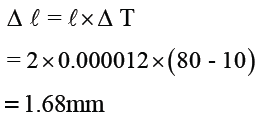QUESTION: 22

Brass rod 20mm in diameter is subjected to tensile load of 40 kN. Extension of rod measured with extension meter is found to be 254 divisions in 200mm. If each division is equal to 0.001mm, the elastic modulus of brass is :-

Solution: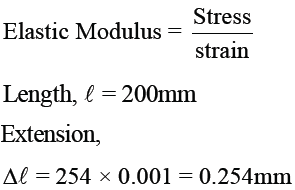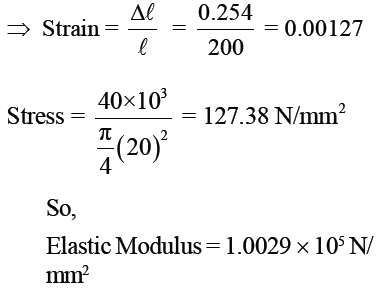QUESTION: 23

The bend ABC shown in the figure carries a concentrated vertical load P at A. The horizontal deflection at A is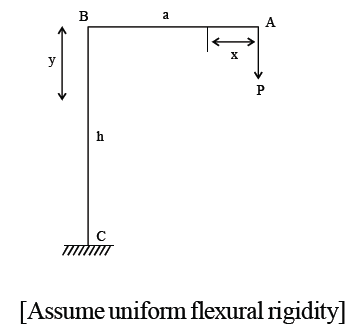Solution: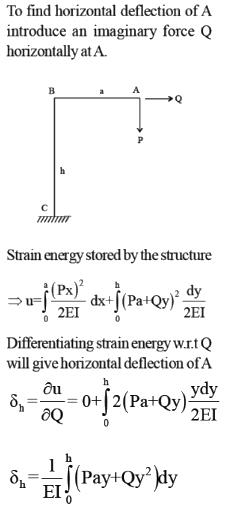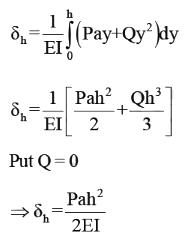QUESTION: 24

For the frame shown in the figure, find the horizontal deflection of the roller end D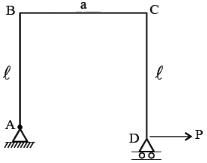Solution: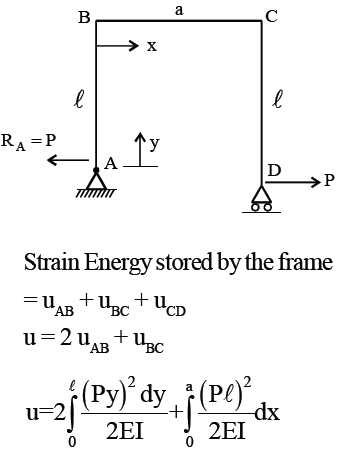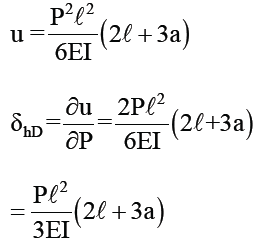QUESTION: 25

For the semi-circular arch or radius R as shown in the figure, the horizontal deflection at roller and B is.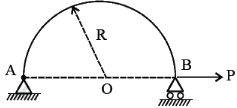Solution: